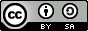## Friday, March 16, 2012

### How to model storage growth for an organization: An approach.

I have always wondered about how to model storage growth. This post is the beginning of an answer and will be living document on the topic going forward. I will fill in more analysis and revise it as time permits and as I learn more. I don't have time (yet) to do experiments on this proposal.

I have heard the data growth question in various forms:
• How much space will we use next year?
• How much data will we have next year?
• Can you model the growth of our data?
• How much storage should we buy?
• What method can I use for predicting future storage growth (or data growth)?
As often happens in the analysis of systems that change over time, I think the right model lies in solving a relationship of of rates of change (differentials). The most practical application of my proposal may be to take measurements of the data in your organization over time and then determine the your specific model numerically.

### My proposal for modeling storage growth:

Determine the set of data generators (home directories, sandboxes, log files, etc.) in your organization. Determine the growth curve for each data generator and then sum the growth curves.  The total growth curve is the sum of the growth curves of your data generators.

Additionally, I propose that one can determine S(t) determined analytically by first determining the change in the number of each data generator (n) and the change in the average size each generator (g). Then S follows from:

### A practical method for using this proposal:

You should be able to model S(t) directly through observation. Determine all of the data generators in your organization. Record their size over a time period. Find the best fit function for their growth over time.  Sum those best-fit functions to determine your organization's total growth curve, S(t).

Alternatively, measure size of the growth of the number of each data generator (n) and the growth in the size of the average size that each data generator (g) in your organization over time. Find best-fit curves for each n and g. Plug the derivatives of each n and g into the lower relationship above and then integrate to find each s.

This latter technique also gives the avenue for testing this proposal. Determine g and n analytically, and then s for each data generator and then compare to the curve fits for each s, n, and g.

### Details of the analytic approach:

is the total growth of the data in your organization as a function of time.
is the data generated by a particular data generator as a function of time. Data generators are things like home directories, sandboxes, or logs.

I propose that the second derivative of the growth curve of a data generator is equal to the product of the change in the size each data generator instance and the change in the number data generator instances.

where

is the number of data generator instances as a function of time and

is the size of each instance of a data generator as a function of time.

So,

where g and n depend on the nature of the data generator.

### Example: linear home directory growth with linear employee growth

For example for each employee, there exists a home directory. This means that n for home directories will depend on the model that determines the number of employees as a function of time. This model may have a periodic element to account for expansion and contraction due to the business cycle.

In a simple case, the size of a home directory and the number of employees both grow linearly.

Then s for home directories would show quadratic growth of data over time:

Where A and B are measured and C and D depend on the initial average size of a home directory and the initial number of employees.

### Questions for further consideration:

• What are the most common data generators? Certainly home directories, logs, and email accounts - but what else?
• Is there a strict concrete definition of a data generator? If so, what is it? Having one will help us create experiments to test the proposals above.
• What are the shapes of the g functions for common data generators? For example, consider a new non-developer. Their home directory, consisting only of the standard skeleton, starts off at some size K. What is the shape of the growth curve of their home directory? Answering this question will help both those of us modeling storage growth and those of us monitoring storage growth. An out-of-model home directory, for example, could be flagged for investigation.
• What are the shapes of the n functions for common data generators? What are the shapes of the growth curve for the number of employees as a function of time? What is the shape of the growth in the log data generated by a particular server as a function of time?

### Related reading on modeling data growth I've found so far:

-Adam Keck

(a0f29b982)How to model storage growth for an organization: An approach. by Adam Keck is licensed under a Creative Commons Attribution-ShareAlike 3.0 Unported License.
Based on a work at www.bashedupbits.com.
Permissions beyond the scope of this license may be available at adam.s.keck@gmail.com.
Copyright 2012.